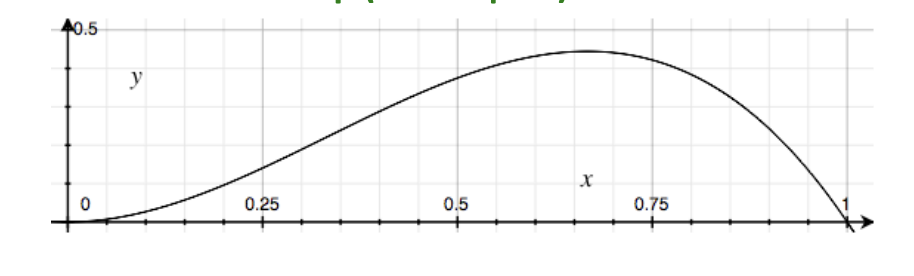Maximizes

Suppose we have a dataset with parameters . For example,

1. We flip a coin three times and get
2. The parameter is the probability that this coin lands heads

The likelihood as a probability mass function . MLE is choosing a that maximizes the likelihood ()In the case above, is

## Notation §

argmin and argmax return the set of parameter values achieving the minimum and maximum values respectively. For example:

We can also show that maximizing the MLE is equivalent to minimizing the negative log-likelihood. That is,

This is true because logarithm is strictly monotonic so the location of the maximum doesn’t change if we take the logarithm. Changing the sign flips the max to the min.

This is typically easier to compute as it turns a product of probability into a sum.

## Generative vs Discriminative §

• Discriminative maximizes
• Least squares, robust linear regression, logistic regression fall under this category
• We don’t model X so we can use complicated features
• Generative maximizes

## Relation between loss functions §

### Least squares (squared L2-loss of residuals) §

If we let the likelihood function of the labels be Gaussian:

Then the MLE of is the minimum of

### Absolute error (L1-loss of residuals) §

If we let the likelihood function of the labels be Laplacian:

Then the MLE of is the minimum of

### Logistic loss §

is the sigmoid function . If we let the likelihood function of the labels be

Then the MLE of is the NLL, which we can show to be equivalent to the logistic loss

Last part is true because of log rules ().

## Overfitting §

Conceptually, MLE is saying that we should find the that makes have the highest probability given . From No Free Lunch Theorem, we know that there is always a model that performs well for some unlikely . This is overfitting!

We actually want to find the that has the highest probability given the data . For this, we need MAP